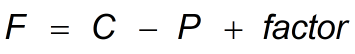Request a Tool

# Gibbs Phase Rule Calculator

Number of degrees of freedom for the phase rule

Number of Moles
0

#### Formula• F = Number of degrees of freedom
• C = Number of components
• P = number of phases in the system
• Factor = is an integer value in the equation that depends on the temperature

#### Defination / Uses

The Gibbs phase rule calculator will help you figure out how many degrees of freedom the phase rule.

### History

The phase rule equation developed by Josiah Willard Gibbs (American theoretical physicist and Yale University professor) in 1875 is stated as above mention in formula.

### Example

NH₄HCO₃(s) <-> NH₃(g) + CO₂(g) + H₂0(g). There are four compounds, one equation, and two conditions in this problem. C = 4 - 1 - 2 = 1, P = 2 The number of degrees of freedom is equivalent to the following: F = 2 + 1 - 2 = 1